``````

# 读取数据

``````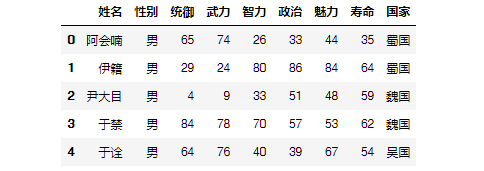``````

#描述性分析
df.describe()

``````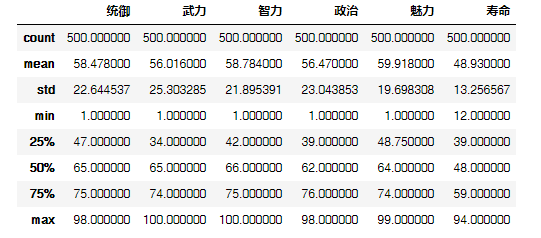• 平均数
1. 简单算数平均数，这里没什么好说的

2. 加权平均数，应用最广泛。这里举个栗子：武力值高不代表带领军队时的战力，不然关羽岂不是无敌，所以这时候用统御能力加权平均更合适。

3. 几何平均数，多用于流程转化中的平均，比如多步骤的转化率求平均值

``````

data = df\['武力'\]
#简单算数平均数
np.average(data)
#加权平均数
np.average(data,weights=df\['统御'\])
#几何平均数
pow(np.prod(data),1/len(data))

``````
• 众数

``````

counts = np.bincount(data)
np.argmax(counts)

``````
• 分位数

``````

# 中位数
np.median(data)
# 四分位数
np.percentile(data, (25, 50, 75), interpolation='midpoint')

``````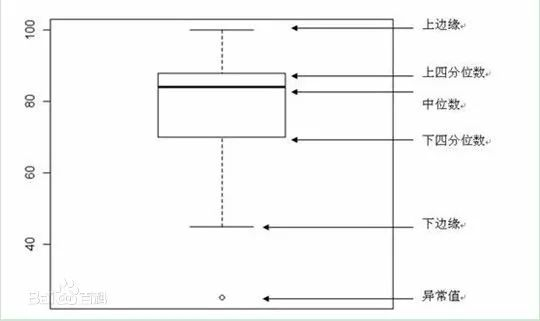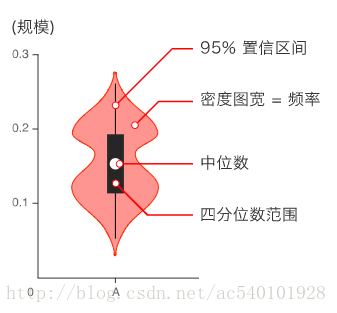• 极差

``````

# 极差
np.max(df\['武力'\])-np.min(df\['武力'\])

``````
• 方差、标准差

``````

# 方差
np.var(df\['武力'\])
# 标准差
np.std(df\['武力'\])

``````
• 平均差

• 异众比率

• 偏态系数

• 峰态系数

``````

#偏度、峰度
from scipy import stats
x = df\_wu\['武力'\]
skew = stats.skew(x)
kurtosis = stats.kurtosis(x)

``````

``````

df\_wei = df.loc\[(df\['国家'\] == '魏国')\]
df\_shu = df.loc\[(df\['国家'\] == '蜀国')\]
df\_wu  = df.loc\[(df\['国家'\] == '吴国')\]
data = df\_wu\['武力'\]
plt.hist(data,20,normed=True,facecolor='g',alpha=0.9)
plt.show()

``````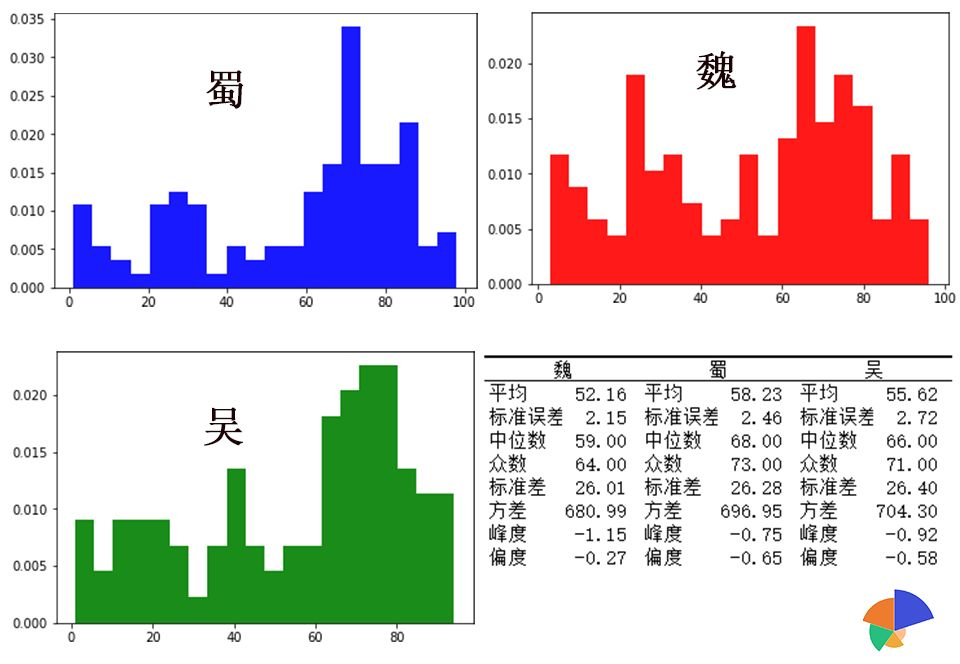1. 从平均值看，蜀国武将的平均武力在三个国家之上

2. 从标准差看，吴>蜀>魏，这说明吴国人物间武力差距更大一些，而魏国人物武力分布较为均匀。

3. 从偏度上看：三国偏态系数均小于0，平均数在众数之左，是一种左偏的分布，又称为负偏。 从上面三个图中也可以看出：其中蜀国的武力分布众数偏在右侧更明显一点，长尾拖在左边。 从峰度上看：三国偏态系数均小于0，均是低峰态，相对来说蜀国人物武力分布较另外两国人物武将武力分布更窄一些。

PS:大家可能注意到求出的偏态系数为负数，这是因为在实际应用中，通常将峰度值做减3处理。

点击左下角原文链接，直接进入知识星球（免费）原贴获取文中涉及的三国数据和ipynb格式的python代码。

或者微信后台回复“统计分析”，也可同样获取。

《数据茶水间》-木东居士

《从零进阶！数据分析的统计基础》

《深入浅出统计学》浅梦一笑
4个月前Aidan075
1年前blmius
1年前
MySQL:[Err] 1292 - Incorrect datetime value: ‘0000-00-00 00:00:00‘ for column ‘CREATE_TIME‘ at row 1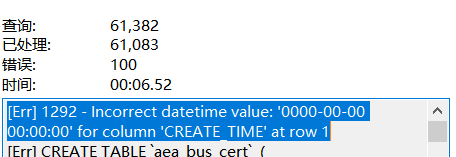Aidan075
1年前

↑一个宝藏公众号，长的好看的人都关注了 简单介绍一下Python吧Python是一种面向对象程序设计语言，由荷兰人吉多·范罗苏姆于19Stella981
1年前
KVM调整cpu和内存Stella981
1年前
Python之time模块的时间戳、时间字符串格式化与转换
Python处理时间和时间戳的内置模块就有time，和datetime两个，本文先说time模块。关于时间戳的几个概念时间戳，根据1970年1月1日00:00:00开始按秒计算的偏移量。时间元组（struct_time），包含9个元素。 time.struct_time(tm_yWesley13
1年前
MySQL查询按照指定规则排序
1.按照指定(单个)字段排序selectfromtable_nameorderiddesc;2.按照指定(多个)字段排序selectfromtable_nameorderiddesc,statusdesc;3.按照指定字段和规则排序selecStella981
1年前
Angular material matWesley13
1年前
MySQL部分从库上面因为大量的临时表tmp_table造成慢查询helloworld_34035044
6个月前

​在我们用皕杰报表工具设计填报报表时，如何在新增行里自动增加id呢？能新增整数排序id吗？目前可以在新增行里自动增加id，但只能用uuid函数增加UUID编码，不能新增整数排序id。uuid函数说明：获取一个UUID，可以在填报表中用来创建数据ID语法：uuid()或uuid(sep)参数说明：sep布尔值，生成的uuid中是否包含分隔符''，缺省为Aidan075
Lv1

128

1

6